# Position control exampleusing SimpleFOCShield

For this BLDC motor position control example we are going to be using this hardware:

Arduino UNOArduino SimpleFOCShieldAMT 103 encoderIPower GBM4108H-120T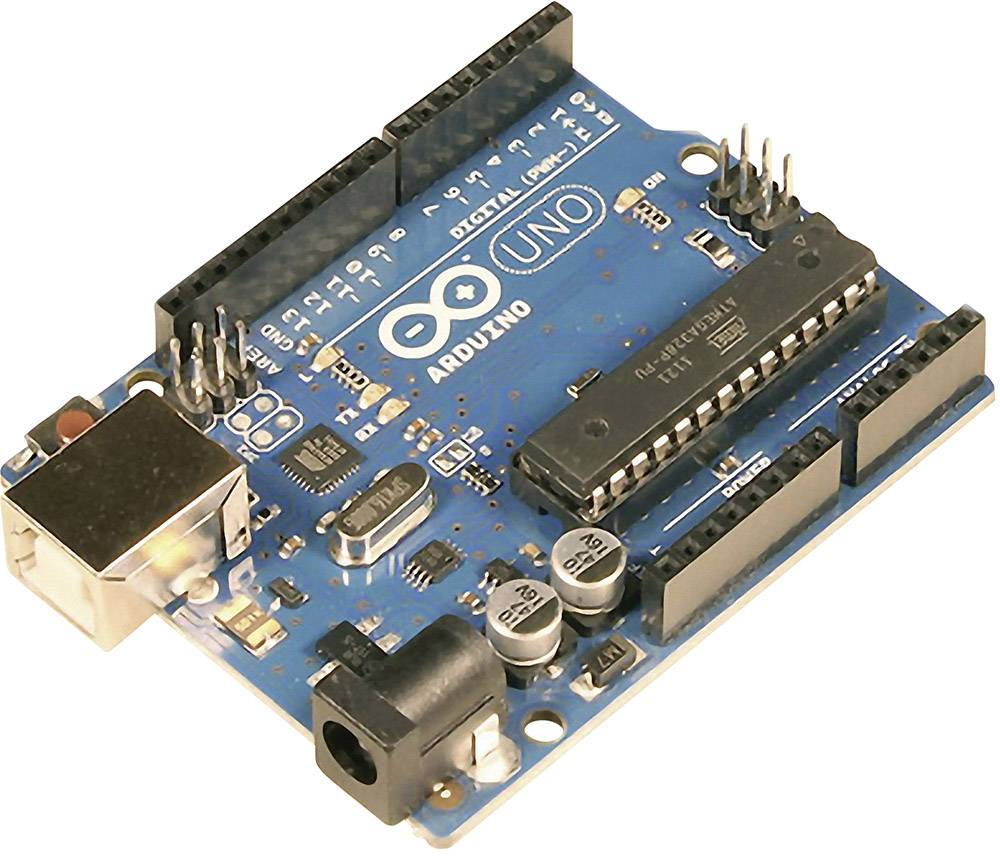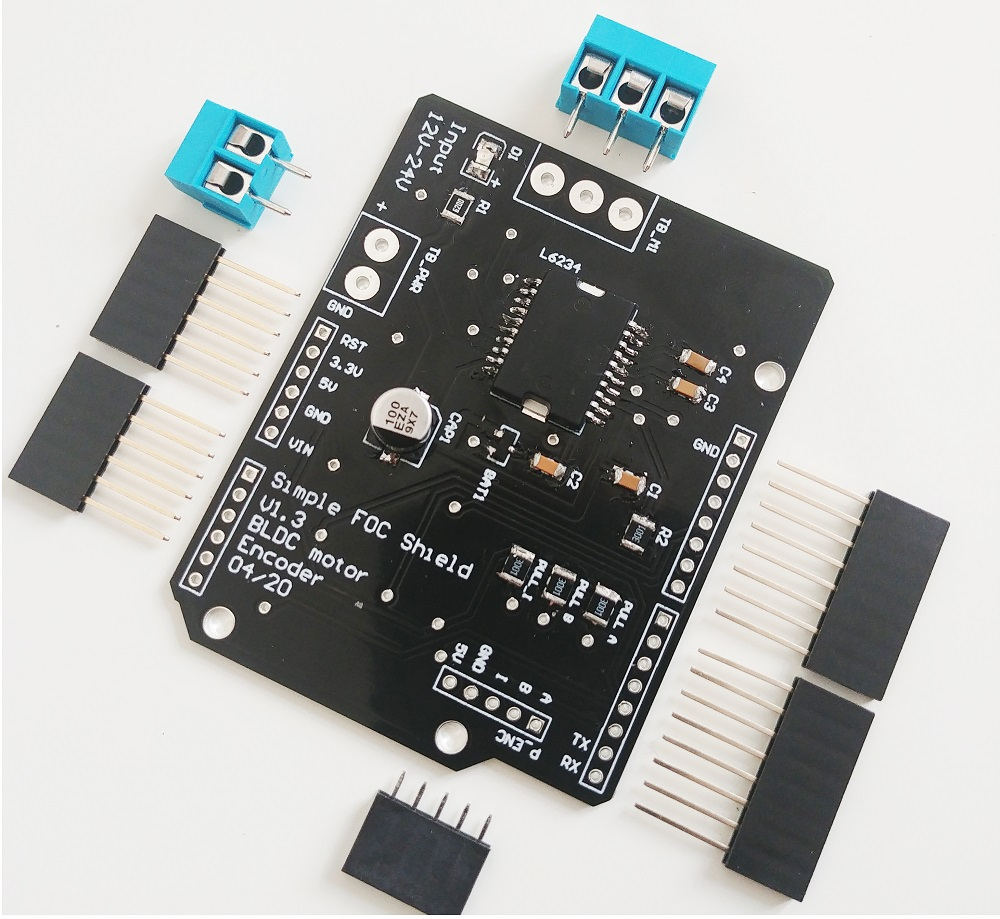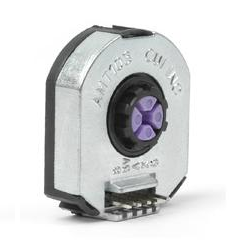# Connecting everything together

For a bit more in depth explanation of Arduino UNO and SimpleFOCShield connection please check the connection examples.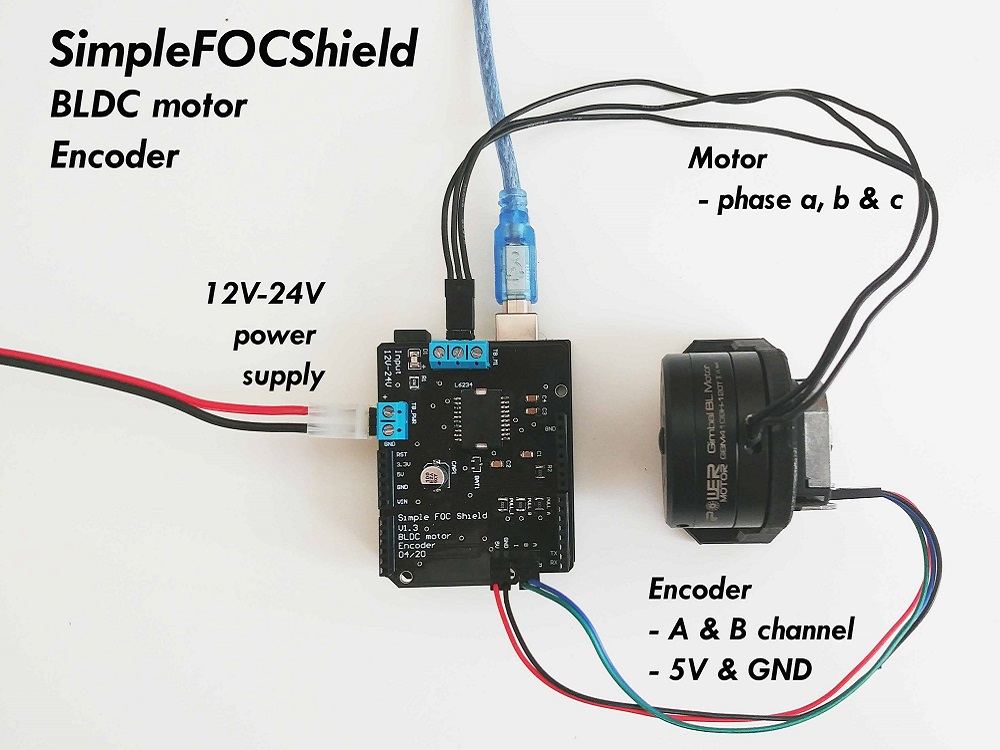## Encoder

• Channels A and B are connected to the encoder connector P_ENC, terminals A and B.

## Motor

• Motor phases a, b and c are connected directly the motor terminal connector TB_M1

### Small motivation :D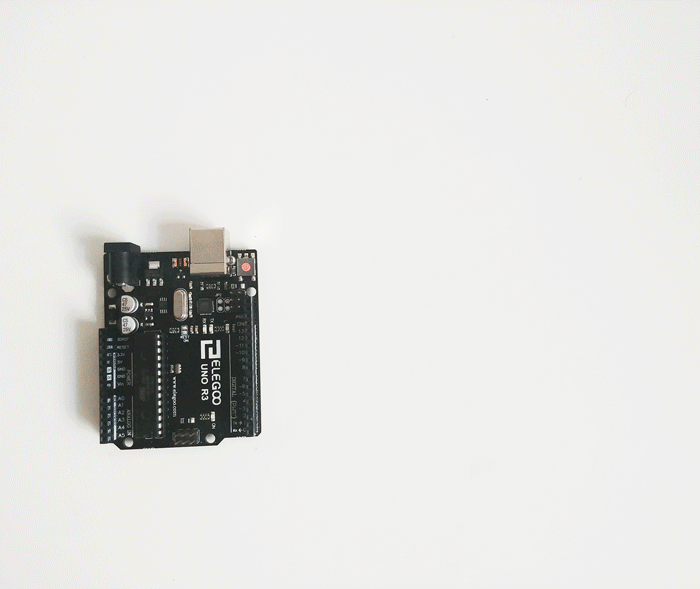# Arduino code

Let’s go through the full code for this example and write it together. First thing you need to do is include the SimpleFOC library:

#include <SimpleFOC.h>


Make sure you have the library installed. If you still don’t have it please check the get started page

## Encoder code

First we define the Encoder class with the A and B channel pins and number of impulses per revolution.

// define Encoder
Encoder encoder = Encoder(2, 3, 2048);


Then we define the buffering callback functions.

// channel A and B callbacks
void doA(){encoder.handleA();}
void doB(){encoder.handleB();}


In the setup() function we initialize the encoder and enable interrupts:

// initialize encoder hardware
encoder.init();
// hardware interrupt enable
encoder.enableInterrupts(doA, doB);


And that is it, let’s setup the motor.

For more configuration parameters of the encoders please check the Encoder class docs.

## Motor code

First we need to define the BLDCMotor class with the number od pole pairs (11)

// define BLDC motor
BLDCMotor motor = BLDCMotor(11);

If you are not sure what your pole pairs number is please check the find_pole_pairs.ino example.

Next we need to define the BLDCDriver3PWM class with the PWM pin numbers of the motor and the driver enable pin

// define BLDC driver
BLDCDriver3PWM driver = BLDCDriver3PWM(9, 10, 11, 8);


Then in the setup() we configure first the voltage of the power supply if it is not 12 Volts and init the driver.

// power supply voltage
// default 12V
driver.voltage_power_supply = 12;
driver.init();


Then we tell the motor which control loop to run by specifying the motor.controller variable.

// set control loop type to be used
// MotionControlType::torque
// MotionControlType::velocity
// MotionControlType::angle
motor.controller = MotionControlType::angle;


Now we configure the velocity PI controller parameters

// velocity PI controller parameters
// default P=0.5 I = 10
motor.PID_velocity.P = 0.2;
motor.PID_velocity.I = 20;
// jerk control using voltage voltage ramp
// default value is 300 volts per sec  ~ 0.3V per millisecond
motor.PID_velocity.output_ramp = 1000;

//default voltage_power_supply
motor.voltage_limit = 6;


Additionally we can configure the Low pass filter time constant Tf

// velocity low pass filtering
// default 5ms - try different values to see what is the best.
// the lower the less filtered
motor.LPF_velocity.Tf = 0.01;


Finally we configure position P controller gain and the velocity limit variable.

// angle P controller
// default P=20
motor.P_angle.P = 20;
//  maximal velocity of the position control
// default 20
motor.velocity_limit = 4;


Next we connect the encoder and the driver to the motor, do the hardware init and init of the Field Oriented Control.

// link the motor to the sensor
// link the motor to the driver

// initialize motor
motor.init();
// align encoder and start FOC
motor.initFOC();


The last peace of code important for the motor is of course the FOC routine in the loop function.

void loop() {
// iterative FOC function
motor.loopFOC();

// iterative function setting and calculating the angle/position loop
// this function can be run at much lower frequency than loopFOC function
motor.move(target_angle);
}


That is it, let’s see the full code now!

For more configuration parameters and control loops please check the BLDCMotor class doc.

## Full Arduino code

To the full code I have added a small serial commander interface, to be able to change position/angle target value in real time.

#include <SimpleFOC.h>

// init BLDC motor
BLDCMotor motor = BLDCMotor( 11 );
// init driver
BLDCDriver3PWM driver = BLDCDriver3PWM(9, 10, 11, 8);
//  init encoder
Encoder encoder = Encoder(2, 3, 2048);
// channel A and B callbacks
void doA(){encoder.handleA();}
void doB(){encoder.handleB();}

// angle set point variable
float target_angle = 0;
// commander interface
Commander command = Commander(Serial);
void onTarget(char* cmd){ command.scalar(&target_angle, cmd); }

void setup() {

// initialize encoder hardware
encoder.init();
// hardware interrupt enable
encoder.enableInterrupts(doA, doB);
// link the motor to the sensor

// power supply voltage
// default 12V
driver.voltage_power_supply = 12;
driver.init();
// link the motor to the driver

// set control loop to be used
motor.controller = MotionControlType::angle;

// controller configuration based on the control type
// velocity PI controller parameters
// default P=0.5 I = 10
motor.PID_velocity.P = 0.2;
motor.PID_velocity.I = 20;
// jerk control using voltage voltage ramp
// default value is 300 volts per sec  ~ 0.3V per millisecond
motor.PID_velocity.output_ramp = 1000;

//default voltage_power_supply
motor.voltage_limit = 6;

// velocity low pass filtering
// default 5ms - try different values to see what is the best.
// the lower the less filtered
motor.LPF_velocity.Tf = 0.01;

// angle P controller
// default P=20
motor.P_angle.P = 20;
//  maximal velocity of the position control
// default 20
motor.velocity_limit = 4;

// initialize motor
motor.init();
// align encoder and start FOC
motor.initFOC();

// monitoring port
Serial.begin(115200);
Serial.println("Set the target angle using serial terminal:");
_delay(1000);
}

void loop() {
// iterative FOC function
motor.loopFOC();

// function calculating the outer position loop and setting the target position
motor.move(target_angle);

// user communication
command.run();
}